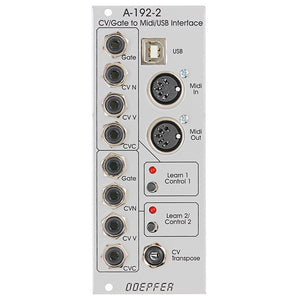# Doepfer - A-192-2 Dual CV/Gate to Midi/USB Interface

• \$149.00

Module A-192-2 contains two independent CV/Gate-to-Midi/USB interfaces.

For each of the two sub-units these inputs are available:

• Gate Input (min. +5V)
• CVN Input (defines the Midi note number), 1V/octave standard, range 0...+10V (i.e. 10 octaves)
• CVV Input (defines the velocity value assigned to the Midi note message), can be used alternatively for Midi volume (CC#7), range 0...+5V
• CVC Input (free assignable to any Midi control change number), range 0...+5V

For both sub-units a common CV Transpose input is available (1V/octave, range 0...+10V). The voltage applied to this input is added internally to CVN before the Midi note number is generated. It can be used e.g. to transpose two sequences simultaneously by one voltage.

Whenever the rising edge of the Gate input is recogized a Midi note on message is generated. The note number corresponds to the sum of the voltages applied to the CVN input and the common CV Transpose Input.

In Velocity mode the voltage applied to the CVV input is used to define the velocity data of the Midi note on message (in Volume mode a fixed velocity value 100 is used). As soon as the falling edge of the Gate input is recognized the corresponding Midi note off message is generated (i.e. with the same note number as the preceding Midi note on message). The voltages at the CVN and CV Transpose inputs during the falling edge of the Gate do not have an effect on the note number of the note off message ! In any case the note number of the preceding note on message is taken. Otherwise hanging Midi notes would occur !

The CVC voltage is permanently converted into the corresponding Midi control change message, as well as the CVV voltage provided that the Volume mode is chosen. The difference between Velocity mode and Volume mode is the function of the voltage applied to CVV. In Velocity mode the voltage CVV is used to define the velocity value of the corresponding Midi note on message and is measured only during the rising edge of the Gate input. In Volume mode the CVV voltage is permanently converted into Midi Volume messages (Midi control change #7).

Power consumption: 70mA at +12V and 20mA at -12V

Depth: 55mm

HP : 10